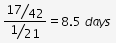# A can do a piece of work in 14 days, while B can do in 21 days

A can do a piece of work in 14 days, while B can do in 21 days.They begin together. But ,3 days before the completion of the work, A leaves off. Find the total number of days taken to complete the work.

If A can do the wrok in 14 days, his work per day = 1/14
if B can do the work in 21 days, his work per day = 1/21
if A and B work together, work per day = (1/14)+(1/21) = 5/42
Hence work will be completed, if and B work together = 42/5 = [ 8+(2/5) ] days.
If A leaves 3 days before completion of work, hence they worked together for 5 days.
Hence completed work in 5 days = 5×(5/42) = 25/42
remaining work = 1 - (25/42) = 17/42
Hence no. of days required for B to complete =